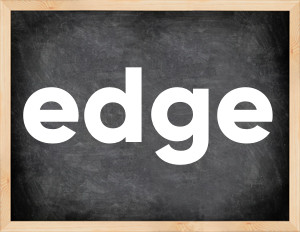# Edge past tenseThe English verb 'edge' is pronounced as [edʒ].
Related to: regular verbs.
3 forms of verb edge: Infinitive (edge), Past Simple - (edged), Past Participle - (edged).

## Here are the past tense forms of the verb edge

👉 Forms of verb edge in future and past simple and past participle.
❓ What is the past tense of edge.

## Edge: Past, Present, and Participle Forms

Base Form Past Simple Past Participle
edge [edʒ]

edged [edʒd]

edged [edʒd]

## What are the 2nd and 3rd forms of the verb edge?

🎓 What are the past simple, future simple, present perfect, past perfect, and future perfect forms of the base form (infinitive) 'edge'?

### Learn the three forms of the English verb 'edge'

• the first form (V1) is 'edge' used in present simple and future simple tenses.
• the second form (V2) is 'edged' used in past simple tense.
• the third form (V3) is 'edged' used in present perfect and past perfect tenses.

## What are the past tense and past participle of edge?

The past tense and past participle of edge are: edge in past simple is edged, and past participle is edged.

### What is the past tense of edge?

The past tense of the verb "edge" is "edged", and the past participle is "edged".

### Verb Tenses

Past simple — edge in past simple edged (V2).
Future simple — edge in future simple is edge (will + V1).
Present Perfect — edge in present perfect tense is edged (have/has + V3).
Past Perfect — edge in past perfect tense is edged (had + V3).

### edge regular or irregular verb?

👉 Is 'edge' a regular or irregular verb? The verb 'edge' is regular verb.

## Examples of Verb edge in Sentences

•   The blue square was edged by trees (Past Simple)
•   She tried to edge away until she passed the crowd (Past Simple)
•   The city park was edged with bushes. (Past Simple)
•   Glory inflamed their courage, freedom edged their swords. (Past Simple)
•   The piercing chill of the night wind was edged with sea salt. (Past Simple)
•   I had almost won the race when suddenly a guy edged me. (Past Simple)
•   My sister is sort of edged. (Present Simple)
•   In our company, profit have edged up. (Present Perfect)
•   The tablecloth was edged with a hand-woven pattern. (Past Simple)
•   She slowly edged away from him. (Past Simple)

Along with edge, words are popular craft and radiate.

Verbs by letter: , , , , , , , , , , , , , , , , , , , , , , , , .